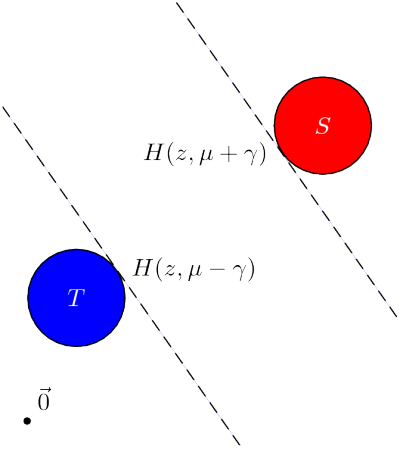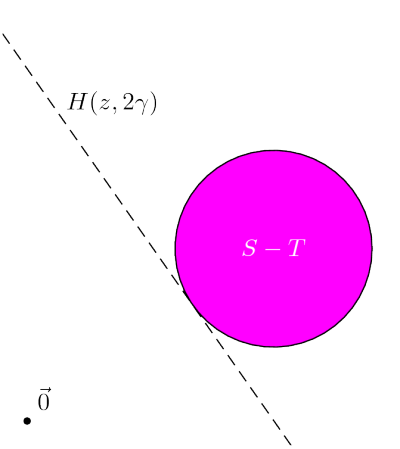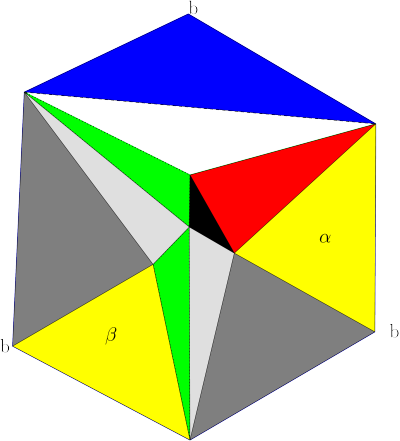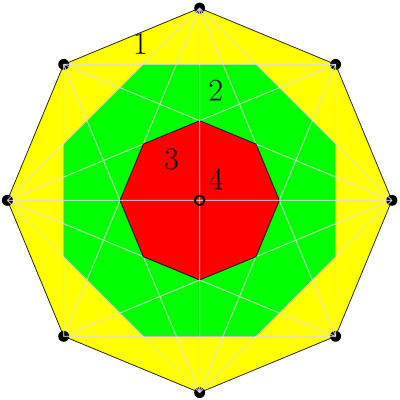UNB/ CS/ David Bremner/ research/ snapshot

## Snapshots From Current Research

November 21, 2017

### 1 Equality, Subtraction, and the Minkowski Difference

One of the first observations one makes in thinking about whether two sets of points S and T are separable by a hyperplane is that one can reduce this to the question of whether the origin is separable from the “Minkowski Difference” S -T = {s-t : s S,t T}. The same sort of elementary linear algebra shows that you can separate S and T with a certain margin 2γ if and only if you can separate the origin from S - T with margin 2γEPSEPS

### 2 Triangulation and Symmetry

The notion of degeneracy is a pervasive one in geometric computation. In general, it tends to mean the existence of more than the minimal possible number of incidences. When computing convex hulls, this means more than d points on a facet. The notion of perturbation or “moving the input a little” is often used as a way of breaking ties, and reducing the total number of incidences. It turns out that perturbation in the context of convex hulls is essentially equivalent to computing a triangulation of the boundary of the convex hull. Unfortunately triangulating the boundary typically breaks up (useful) symmetries. In the figure below, we see the familiar cube cut open along three edges (the three vertices labelled b correspond the cube vertex opposite the center vertex). Before triangulating, there is a natural rotational symmetry to this diagram; afterwards, only those triangles with the same colour can be mapped into each other by rotation. I am currently investigating the trade off between breaking up degeneracy (good) and breaking up symmetry (bad). Some advantage can be gained by (symbolically) perturbing points in the same (sub)-orbit of the symmetry group in a compatible way.EPS

### 3 Halfspace Depth

In non-parametric statistics, no assumption is made about the probability distribution of the population, and the test statistics are usually based on the rank of the data. In multivariate data analysis, every data item consists of several elements (i.e. is an n-tuple). The concept of data depth in non-parametric multivariate statistics is the generalization of the univariate rank method. Given a set S of points, the halfspace depth (or rank) k of point p is defined as the minimum number of points of S excluding p contained in any closed halfspace with p on its boundary. The data with the highest rank is considered the centre of the data set, which best describes the set.EPS